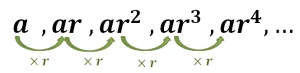# Geometric Formula - Brief Explanation & CalculationsIn mathematics, the term geometric sequence introduces more complicated calculations. But gone are the days when computing this mathematical technique was daunting. Nowadays, you can use a free online geometric sequence calculator to calculate the geometric series of a data set of values. So let us move down to the article and discuss how you can figure it out manually!

Stay Focused!

## What Is Geometric Sequence?

Geometric sequences are those in which the term may be calculated by multiplying the preceding term by a constant quantity known as the common ratio.Mathematically we have:

a, ar, ar2, ar3, ar4, ...

The free geometric sequence calculator also determines the geometric series in the same pattern but in seconds which saves you a lot of time.

Consider a series where the first term is a and the sequence has a common ratio of r. The second term may be obtained by multiplying a by r. Throughout the whole procedure, this process is ongoing. Even the geometric series calculator also follows the same strategy to compute the final results.

Keep in mind that:

• If |r| < 1, the next term is the smaller one
• If |r| > 1, the upcoming term will be greater than the previous one

## Geometric Sequence Formula:

Let us learn how to calculate the nth term of the geometric series that is an!

### Recursive Rule:

This rule can be used to find any missing term of the geometric series. But keep in mind that to apply this rule, you need to keep two things in mind:

• The term before the nth term
• Common ratio to find out the nth term at the end

If you feel any difficulty, you may better use the free geometric sequence calculator by calculator-online.net.

#### Mathematically:

an=an-1*r

Also, if you know the terms an-1& r, you can also resolve for r as under:

r=an/an-1

### Explicit Rule:

For an understanding of this rule, you may need to explore the geometric series first;

a1 = a
a2 = a * r
a3 = a * r * r

...

...

...

If you want to apply this rule to determine the nth term of the sequence, then you need to find the (n-1)th term. This yields the final mathematical statement of the explicit rule as follows:

nth Term = a1rn-1

The calculations may seem complicated but do not worry! The free geometric sequence calculator automatically calculates the series which allows you to understand the concept better and in less time.

## Geometric Progression Properties:

• If b^2=ac, it means that all the three terms a, b, and c are in geometric progression
• The sum and product of two GPs is another GP
• GP can instantly be determined by using the geometric sequence calculator
• In a geometric progression

If three terms are involved, they will be written as

a/r, a, ar

If four terms are involved, they will be written as

a/r3, a/r, ar, ar3

• In a geometric progression, the first term and the last term are equally spaced from one another that can be analyzed through a histogram
• When a GP is multiplied or divided by a non-zero constant, the new sequence also has the same common ratio as the original GP
• The ensuing sequence is also a GP if each term of a GP is raised to the power by the same non-zero quantity

## Use Geometric Sequence Calculator:

This advanced tool that we are talking about includes intelligent functioning that immediately calculates the geometric series of a data set. Due to the accuracy of results provided by this tool, researchers, mathematicians, statisticians, and pupils are highly attracted to it. So if you feel any problem regarding the issue under discussion, you may better subject to this free online calculator.

## Wrapping It Up:

In the following article, we had a brief overview of the geometric sequence and the way you can calculate it better and fast. We hope you will not feel any difficulty regarding calculating geometric sequences now.